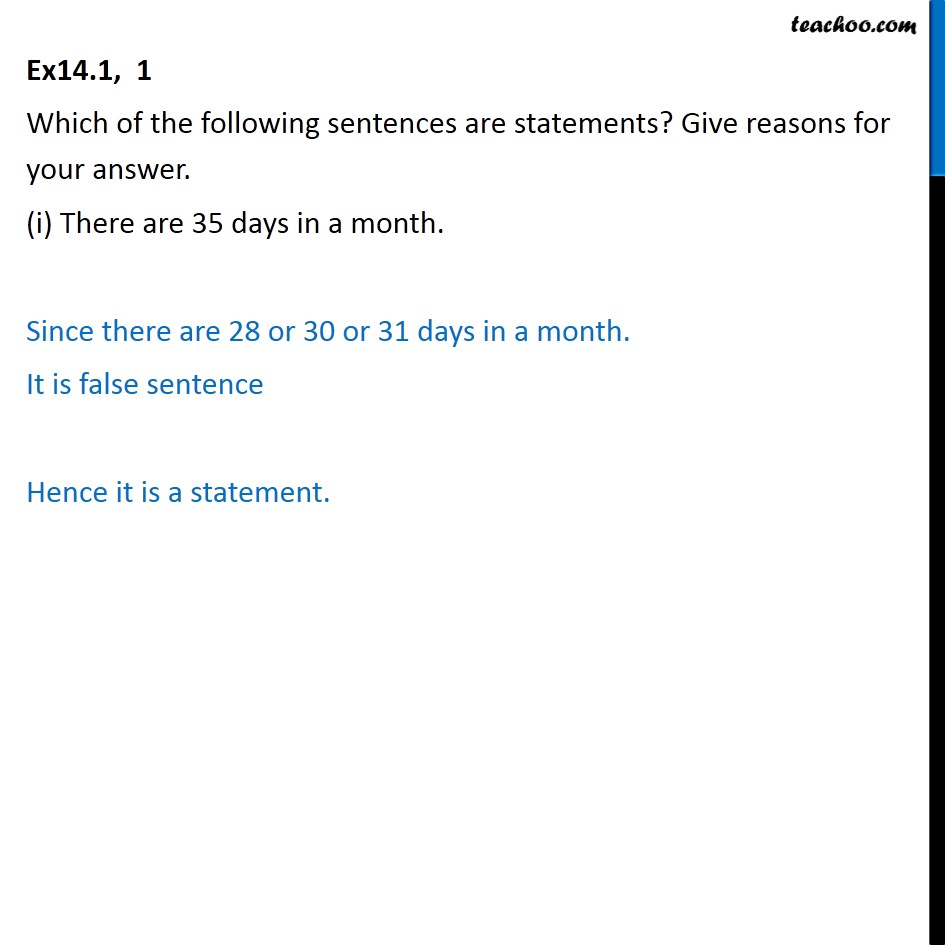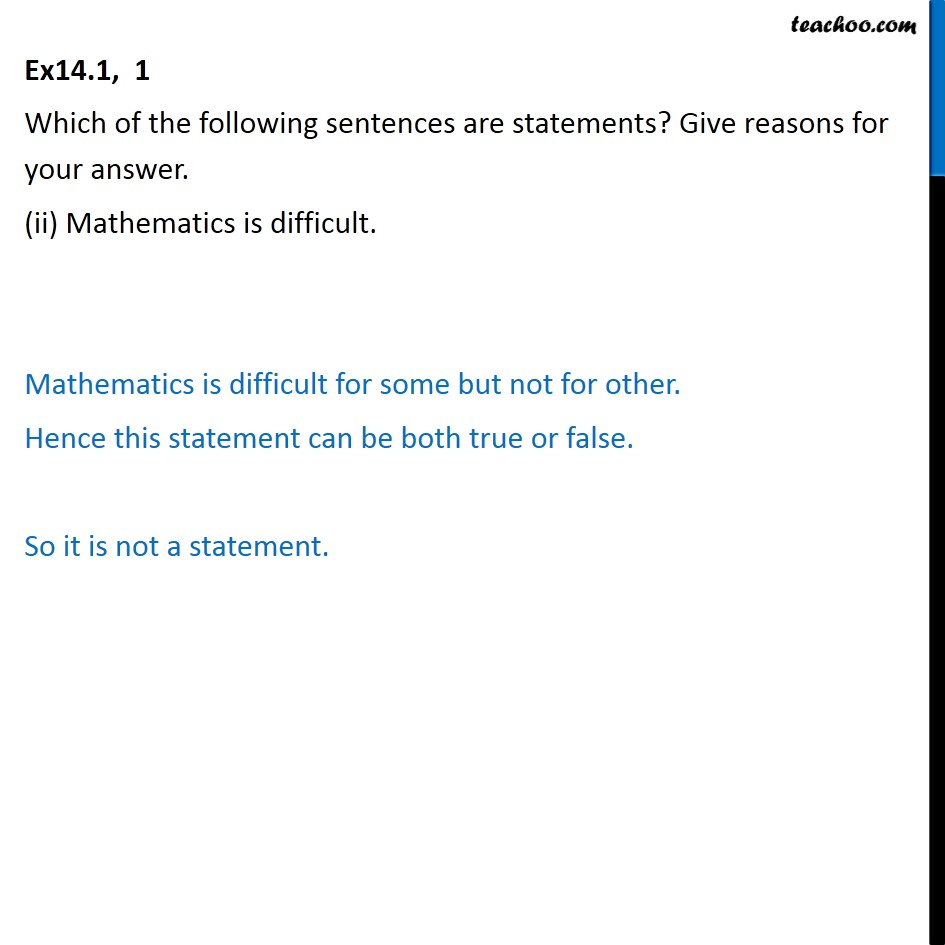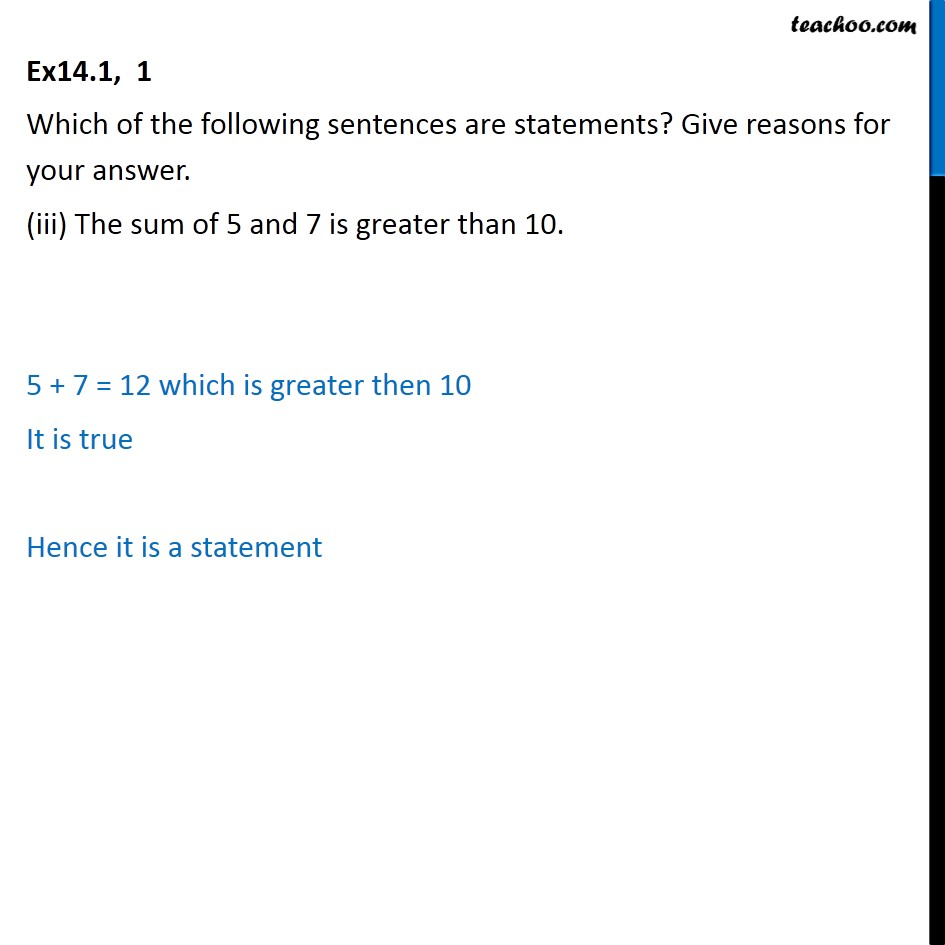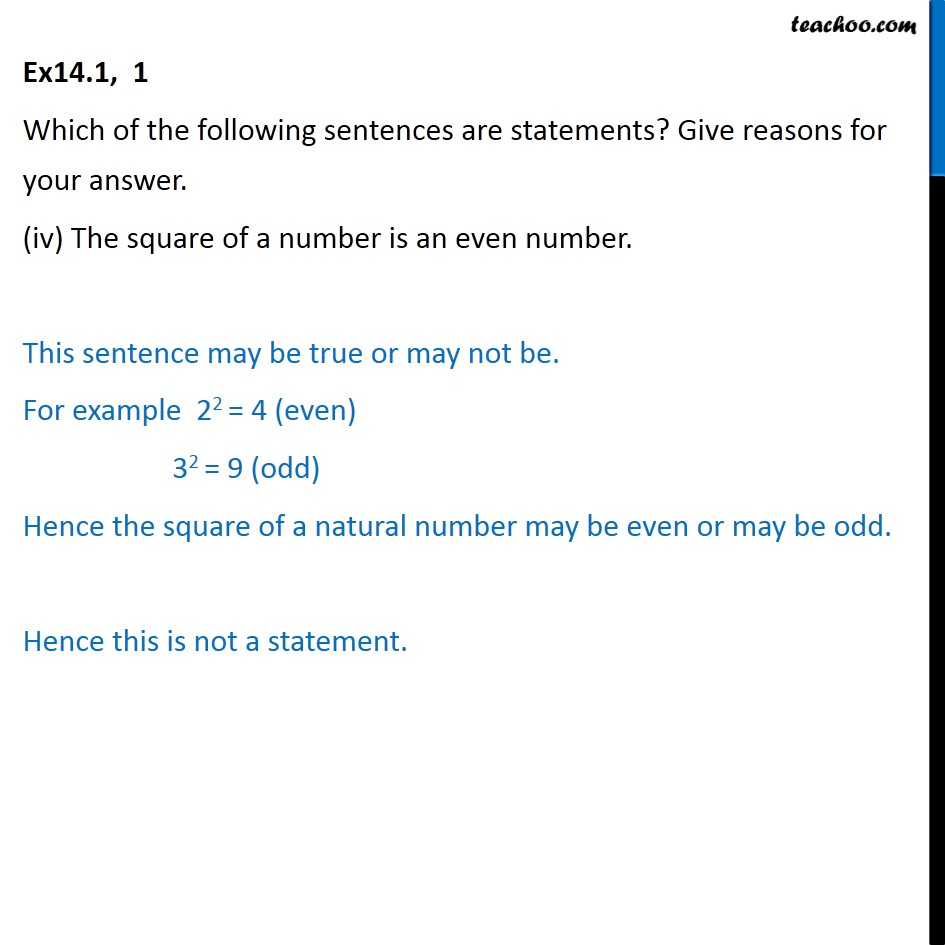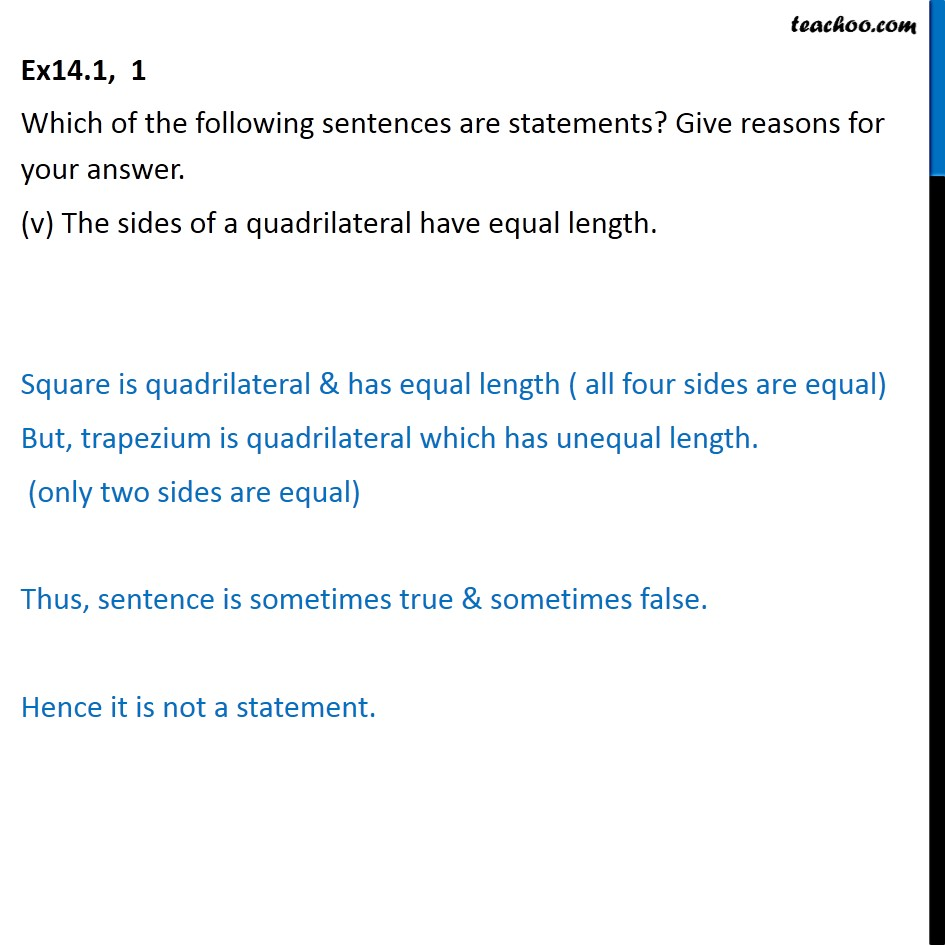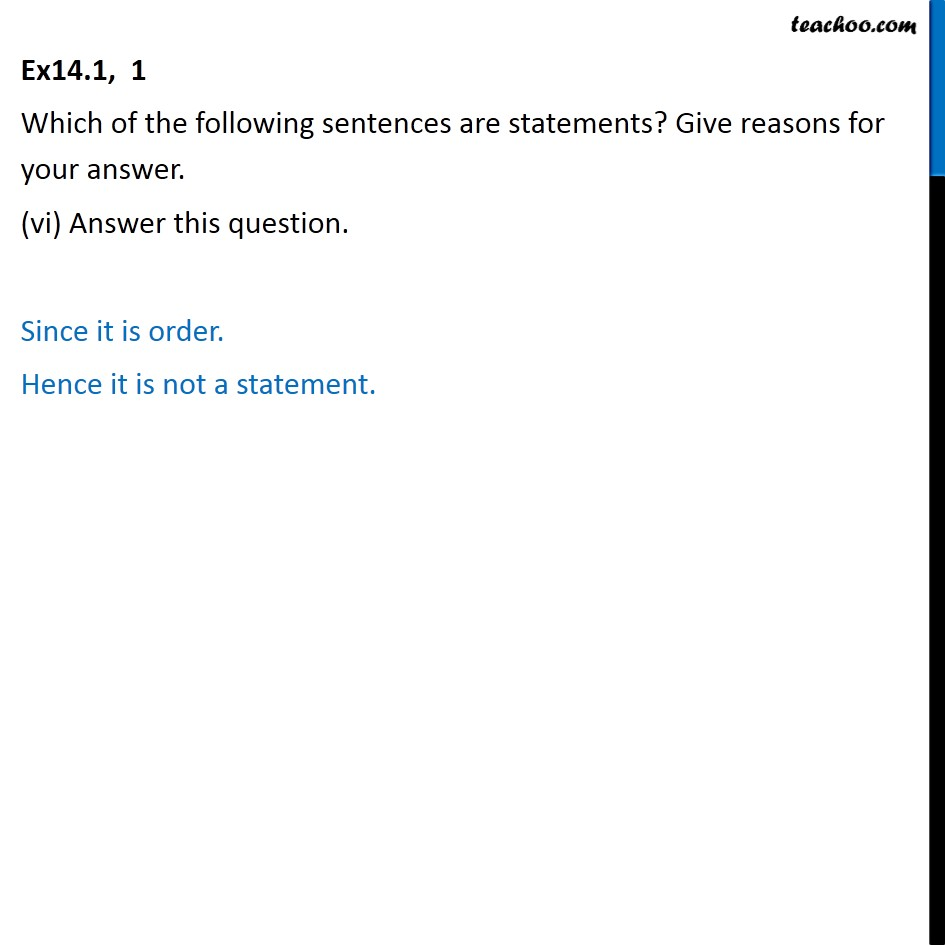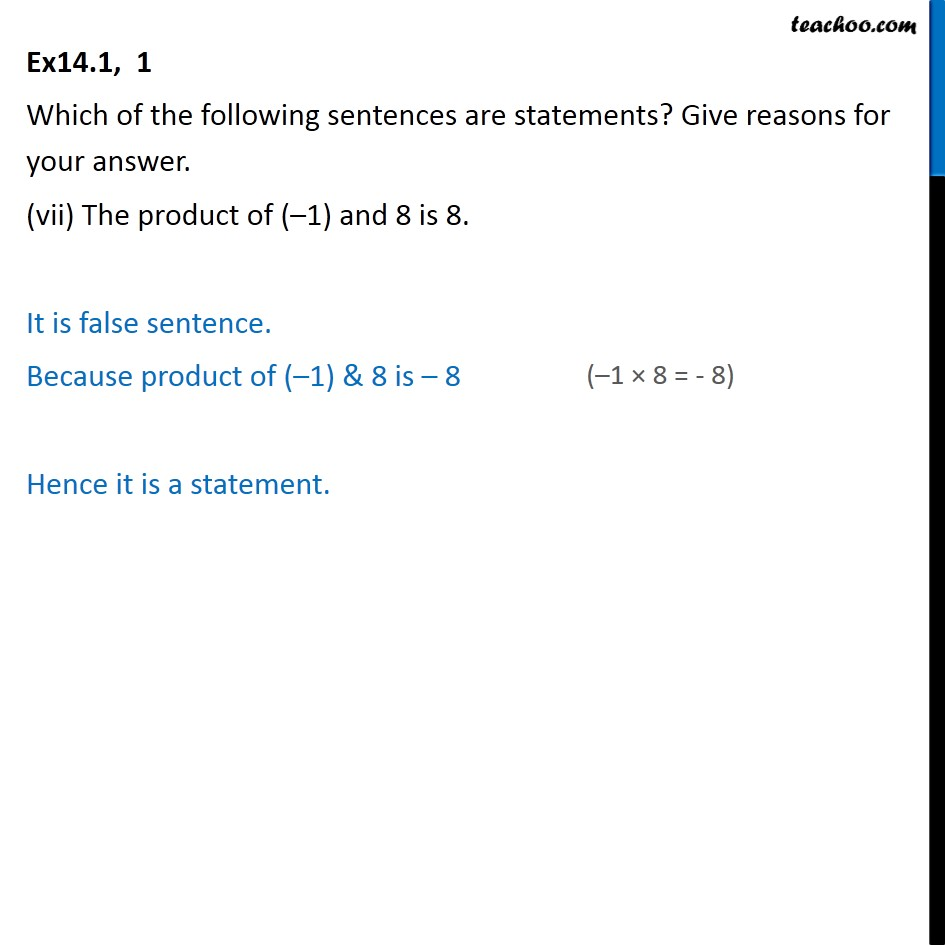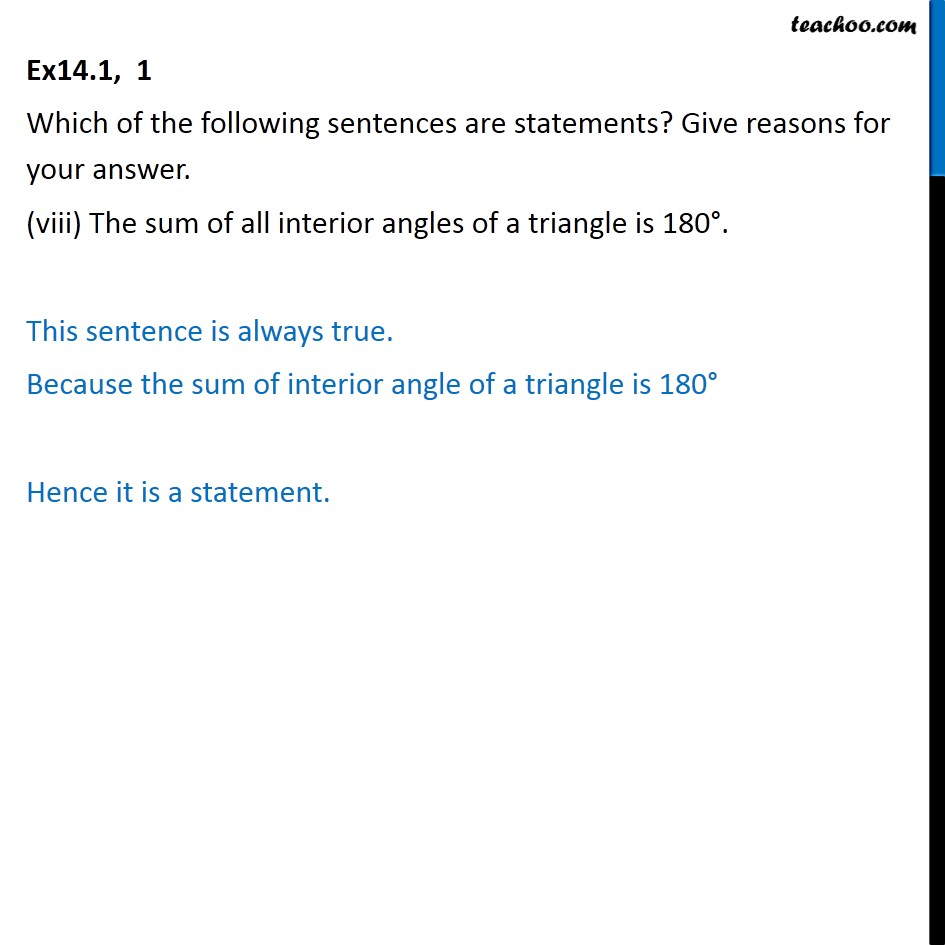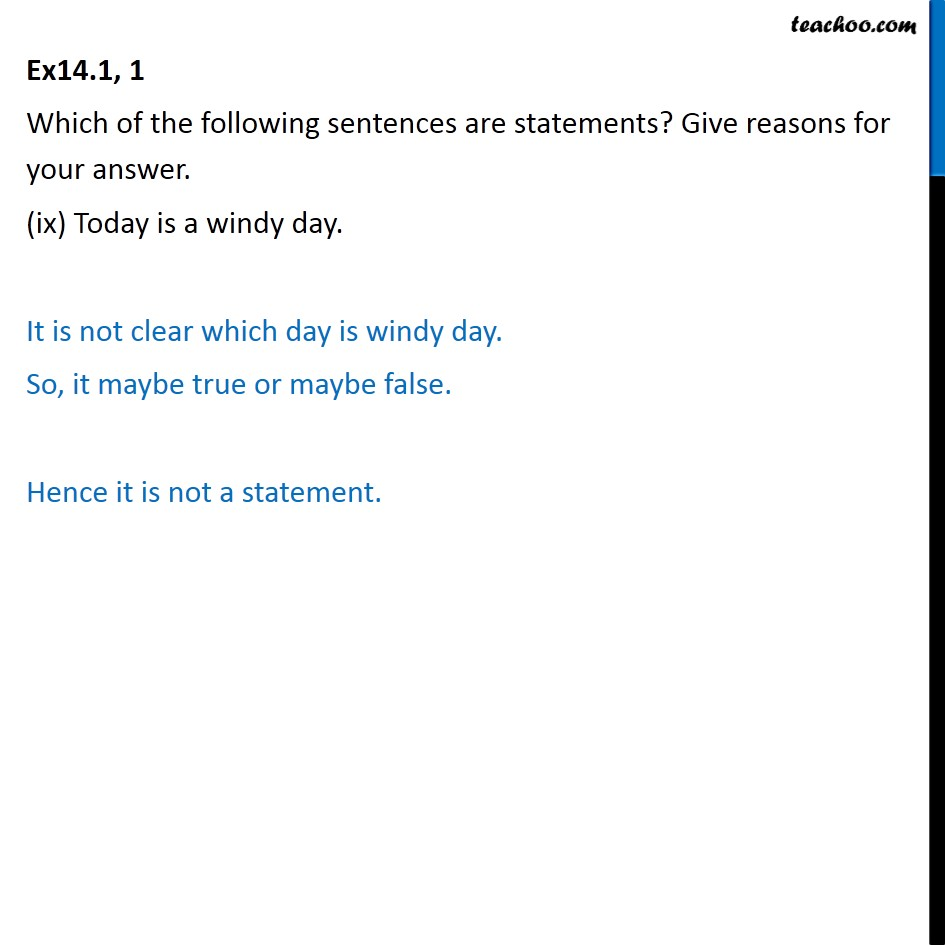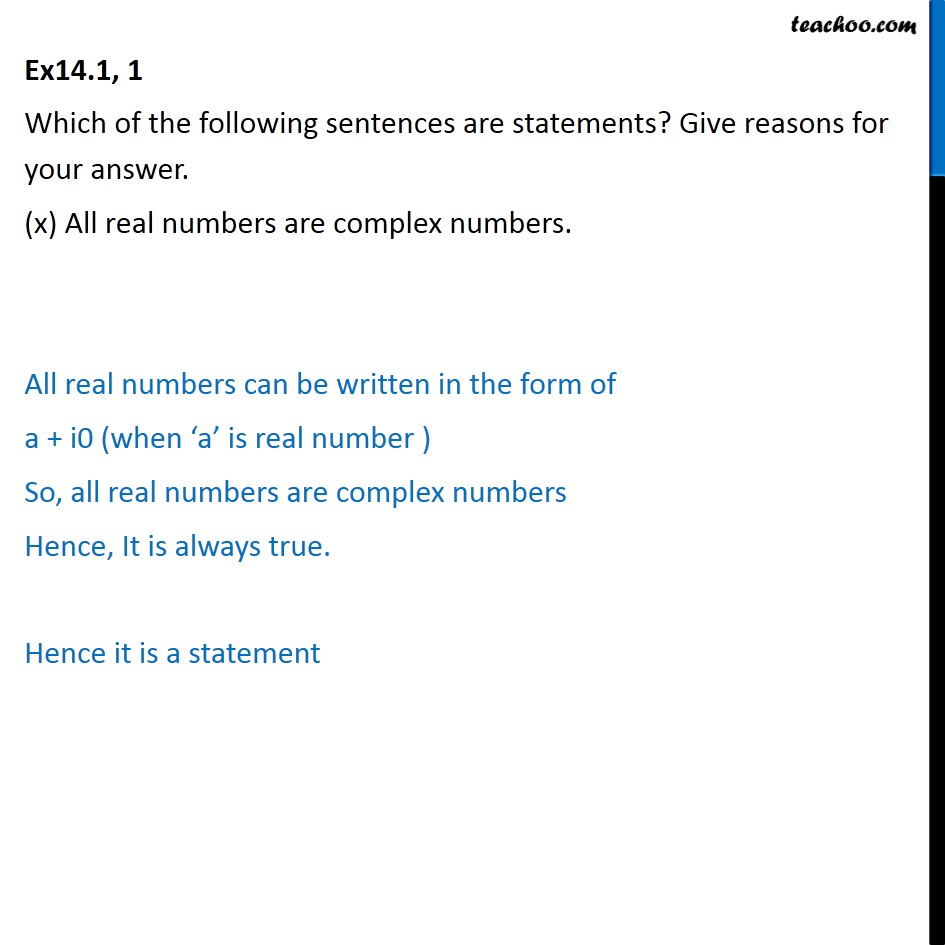1. Chapter 14 Class 11 Mathematical Reasoning
2. Serial order wise
3. Ex 14.1

Transcript

Ex 14.1, 1 Which of the following sentences are statements? Give reasons for your answer. (i) There are 35 days in a month. Since there are 28 or 30 or 31 days in a month. It is false sentence Hence it is a statement. Ex14.1, 1 Which of the following sentences are statements? Give reasons for your answer. (ii) Mathematics is difficult. Mathematics is difficult for some but not for other. Hence this statement can be both true or false. So it is not a statement. Ex14.1, 1 Which of the following sentences are statements? Give reasons for your answer. (iii) The sum of 5 and 7 is greater than 10. 5 + 7 = 12 which is greater then 10 It is true Hence it is a statement Ex14.1, 1 Which of the following sentences are statements? Give reasons for your answer. (iv) The square of a number is an even number. This sentence may be true or may not be. For example 22 = 4 (even) 32 = 9 (odd) Hence the square of a natural number may be even or may be odd. Hence this is not a statement. Ex14.1, 1 Which of the following sentences are statements? Give reasons for your answer. (v) The sides of a quadrilateral have equal length. Square is quadrilateral & has equal length ( all four sides are equal) But, trapezium is quadrilateral which has unequal length. (only two sides are equal) Thus, sentence is sometimes true & sometimes false. Hence it is not a statement. Ex14.1, 1 Which of the following sentences are statements? Give reasons for your answer. (vi) Answer this question. Since it is order. Hence it is not a statement. Ex14.1, 1 Which of the following sentences are statements? Give reasons for your answer. (vii) The product of (–1) and 8 is 8. It is false sentence. Because product of (–1) & 8 is – 8 Hence it is a statement. Ex14.1, 1 Which of the following sentences are statements? Give reasons for your answer. (viii) The sum of all interior angles of a triangle is 180°. This sentence is always true. Because the sum of interior angle of a triangle is 180° Hence it is a statement. Ex14.1, 1 Which of the following sentences are statements? Give reasons for your answer. (ix) Today is a windy day. It is not clear which day is windy day. So, it maybe true or maybe false. Hence it is not a statement. Ex14.1, 1 Which of the following sentences are statements? Give reasons for your answer. (x) All real numbers are complex numbers. All real numbers can be written in the form of a + i0 (when ‘a’ is real number ) So, all real numbers are complex numbers Hence, It is always true. Hence it is a statement

Ex 14.1

About the AuthorDavneet Singh
Davneet Singh is a graduate from Indian Institute of Technology, Kanpur. He has been teaching from the past 9 years. He provides courses for Maths and Science at Teachoo.